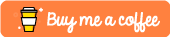# draw-lock-angle

Lock 45, 90, 135, 180... degree when drawing line on Javascript canvas

## Usage no npm install needed!

``````<script type="module">
import drawLockAngle from 'https://cdn.skypack.dev/draw-lock-angle';
</script>``````

# draw-lock-angle

A small library to lock angle when drawing on canvas in JavascriptCheckout the demo https://ittus.github.io/draw-lock-angle/

## How to use

### Install

``````  yarn add draw-lock-angle
``````

### Usage

``````import { calculatePosition } from 'draw-lock-angle'
projection = calculatePosition (newPoint, oldPint, config)
``````

## Config

Prop Type Default Description
isSimple Boolean false If `isSimple` is true, only calculate projection on horizontal line and vertical line. If `isSimple` is false, calculate the nearest projection on 0, 45, 90, 135-degree line
boundary Object. Format: {minX: Number, minY: number, maxX: number, maxY: number} undefined Boundary of calculation

## Development

``````yarn install
``````

### Compiles and hot-reloads for development

``````yarn run serve
``````

### Compiles and minifies for production

``````yarn run build
``````

``````yarn run test
``````yarn run lint